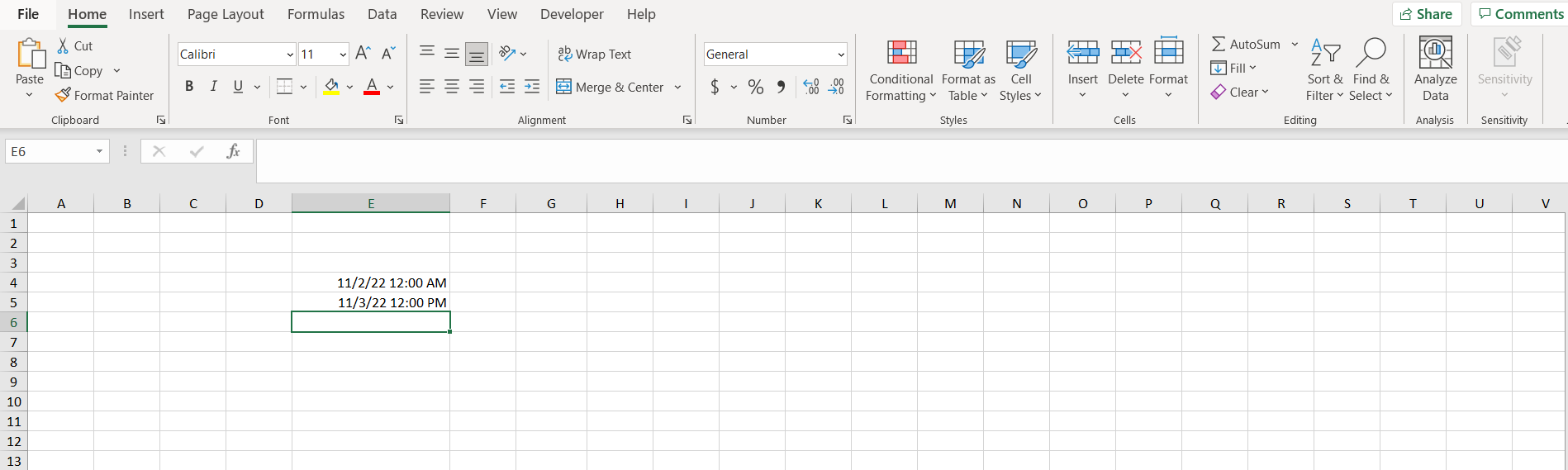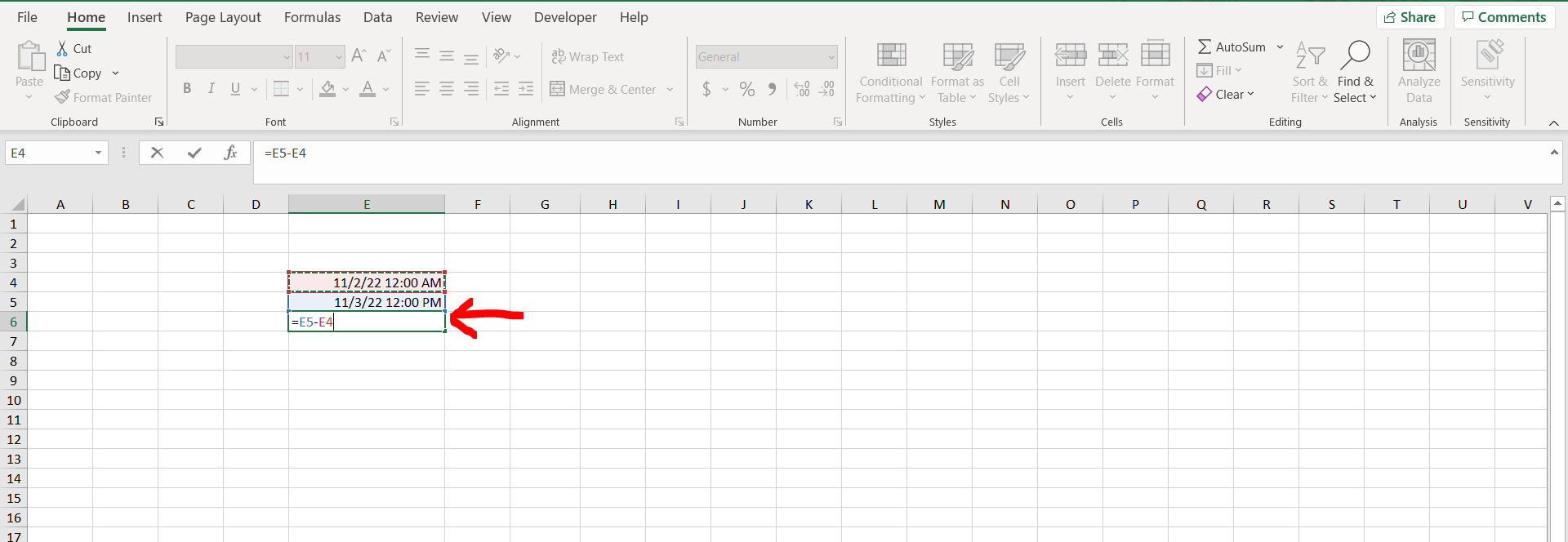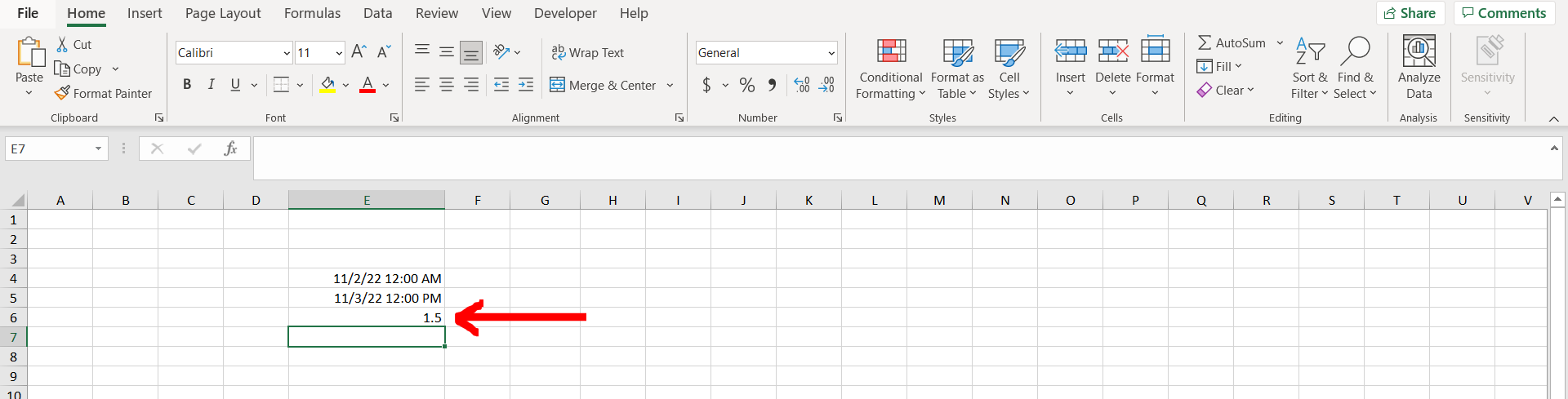# How to calculate difference between two dates and times in ExcelExcel offers a very interesting way to calculate the difference between two dates and times. We can cater to this problem statement by using the “-” operator. We can perform the below mentioned way to calculate difference between two dates and times in excel:

### Step 1 – Excel workbook with 2 dates and times– Open the desired Excel workbook which contains 2 dates and times in different cells

### Step 2 – Subtraction of the cells– Now subtract the 2 cells to get the difference between them in number of days

### Step 3 – Difference obtained– We can see that the difference between the 2 dates and times have been calculated in the number of days..